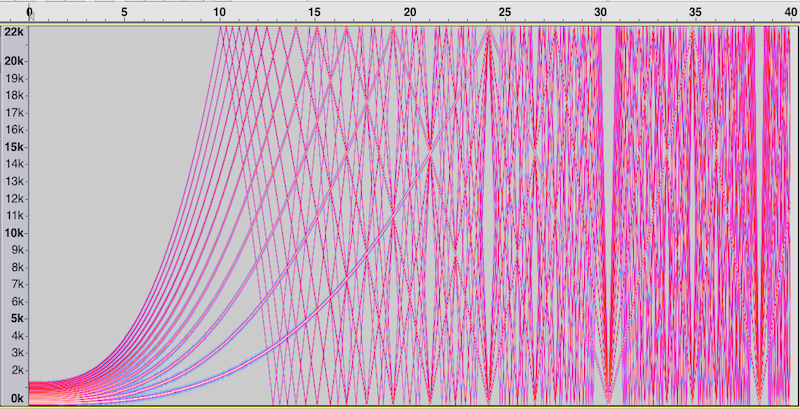# Aliasing Examples

If we try to sample a waveform that contains frequencies higher than the Nyquist frequency (one-half the sampling rate), then aliasing occurs. This means there are audible artifacts that are not actually part of the sampled waveform.

Here is an example. Using a sampling rate of 44100 hz, the following Csound code generates a sound from thirteen sine waves with frequencies that sweep upward from 100 hz to 100000 hz.

```<CsoundSynthesizer>
<CsOptions>
-o aliasing1.wav -W
</CsOptions>
<CsInstruments>
sr = 44100			; audio sampling rate is 44.1 kHz
kr = 44100			; control rate is 44.1 kHz
ksmps = 1			; number of samples in a control period=(sr/kr)
nchnls = 1			; number of channels of audio output
garvbsig  init 0	; make zero at orch init time

instr 2
; p3 is duration
k1 line 0,p3,1
a1 poscil3 10000,100000*k1*k1*k1+100,1
out a1
endin
</CsInstruments>
<CsScore>
; the fundamental waveform: a sum of 13 sine waves with decreasing amplitudes
f 1 0 8192 10 1 0.9 0.8 0.7 0.6 0.5 0.4 0.3 0.2 0.1 0.1 0.1 0.1
; sound will be 40 seconds long
i2 0 40
e
</CsScore>
</CsoundSynthesizer>
```

The above is in csd format: save it all to a single file (like aliasing.csd) and then compile it from the command line with

csound aliasing.csd.

Here is a spectrogram for this sound file:After 10 seconds, the signal contains frequencies higher than 22050 (the Nyquist frequency), and we see the corresponding line in the spectrogram reflect/bounce/fold across the Nyquist value, and we hear the decreasing pitches that result.

Between 10 and 24 seconds, more and more of the waveforms reach the Nyquist frequency and bounce off the Nyquist. At about 24 seconds, all component since waves have reached the Nyquist, and so we should not hear anything at all (all frequencies are beyond the upper frequency limit of our hearing) and yet we do here the aliased signal. So this shows well that the signal is not well represented at this sampling rate.